# Class 12 IP Database Query Using SQL Notes | IP Class 12 SQL Notes

Get well organized Unit-2 Class 12 IP Database Query Using SQL Notes prepared as per CBSE Syllabus cover all topics to score high marks.

#### SQL Functions

• Are subprograms used to manipulate data in different ways as:
• Perform calculations on data
• Modify data items
• Format data items to display
• Summarizes and calculate group of rows
• SQL offers rich set of library of built in functions to perform various operations
• It helps improve the processing, manipulation and maintenance of Database
• There are two types of SQL functions:
• Single Row function
• Multiple Row function#### Single Row Functions

• Also known as Scalar function.
• Return single result for each row of queried table.
• Used for data conversion, modifying and formatting data items.
• Can accept more than one arguments.
• Can be used with Select, Where and Order By clause.
• They are further categorized into
• String/Text Functions
• Numeric Functions
• Date and Time Functions

#### Multiple Row Functions

• It works upon group of rows rather than on single rows.
• It manipulates data of multiple rows at a time and returns aggregated value.
• Used to summarized large volume of data.
• Accept only one argument.
• Can be used with Select statement.
• Also known as Aggregate Function or Group Function.

#### Numeric Functions

Numeric or Mathematical functions are used to perform mathematical calculations on data items. following are the numerical functions which have been discussed in the syllabus:

MOD()
It returns/display remainder after diving a number from another number.
Syntax:
MOD( divident/fieldname, divisor/fieldname)
Example 1:Example 2: For given table ‘Item’ Display remainder after dividing rate by quantity.POW()
It returns/display number raised to given power.
Syntax:
POW( Base/fieldname, Exponent/fieldname)
Example 1: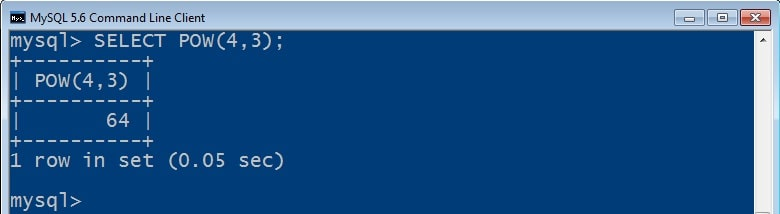ROUND()
It rounds and display a number to the no of decimal places specified.
Syntax:
ROUND( Number/fieldname, no of decimal places)
Example 1: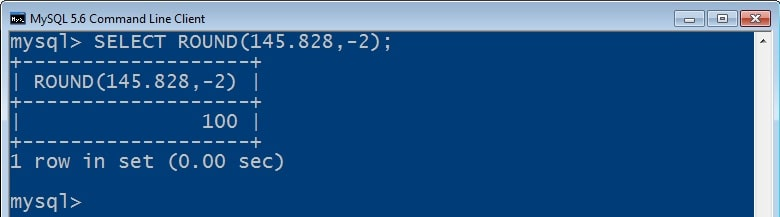Example 2: For given table ‘Club’ Display member names and their fees in round figure.ORSQRT()
It returns and display square root of number passed as argument in it.
Syntax:
SQRT( Number/fieldname)
Example 1: Write SQL command to display square root of 99 rounded by 2 decimal digits.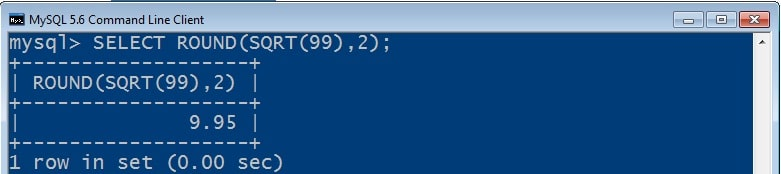#### String/Text Functions

These functions are used with string data items to perfrom various formatting and data conversion operations. following are the string functions which have been discussed in the chapter as per syllabus:

UPPER/UCASE()
It coverts/display character data into uppercase/Capital form.
Syntax:
UPPER(String/Fieldname(s))
UCASE(String/Fieldname(s))
Example 1: For given table ‘Club’ display member names in capital letters whose age not in between 25 to 30yrs.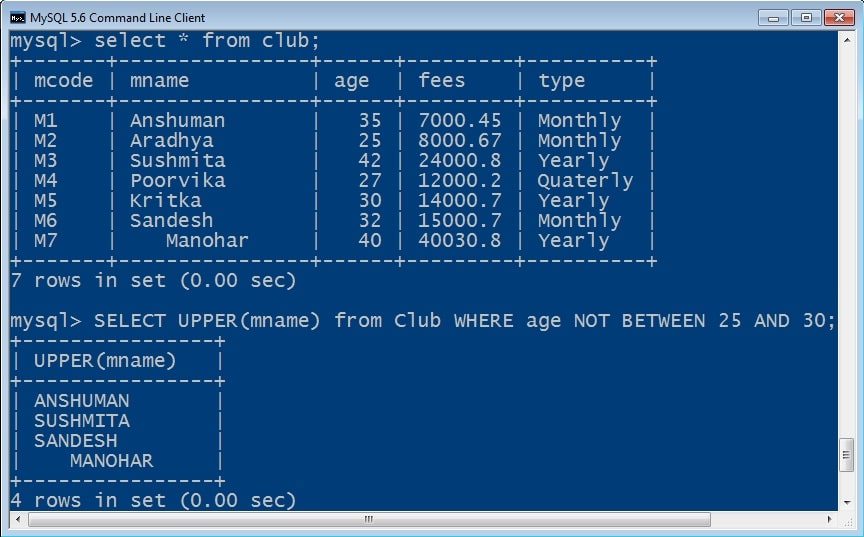LOWER/LCASE()
It coverts/display character data into lowerrcase/Small form.
Syntax:
LOWER(String/Fieldname(s))
LCASE(String/Fieldname(s))
Example 1: For given table ‘Client’ display client names in lowercase who lives in Bombay or Delhi.LENGTH()
It counts and returns/displays total no of characters of given string data.
Syntax:
LENGTH(String/fieldname(s))
Example 1: For given table ‘Client’ display clients whose name consist of 15 characters.LEFT()
It returns/displays leftmost character(s) of string data passed with specified no of characters counting from left.
Syntax:
LEFT(String/fieldname(s), Number of chars)
Example 1:  Predict the output of ‘SELECT UPPER(LEFT(‘techtipnow’,4));’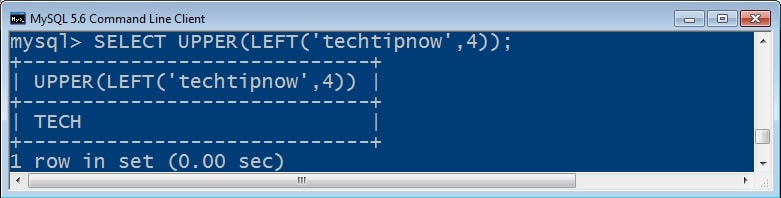Example 2: For given table ‘Library’ Display first 6 character of title which qty is unknown.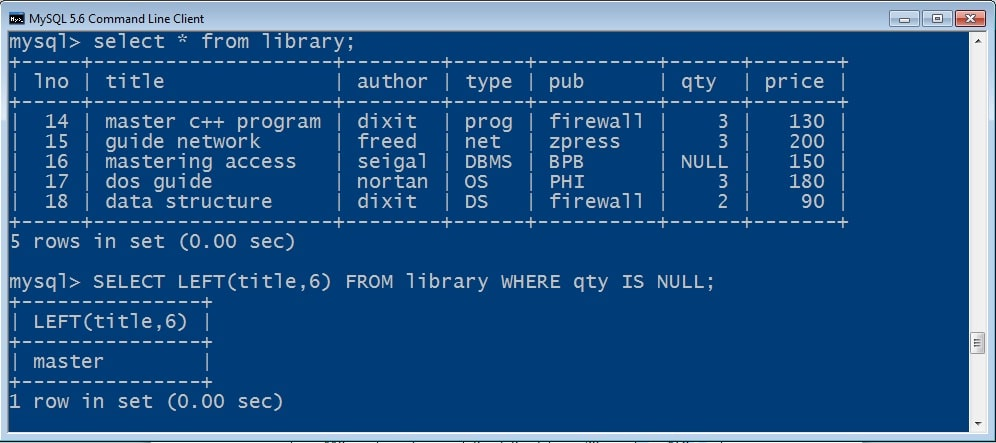RIGHT()
It returns/displays leftmost character(s) of string data passed with specified no of characters counting from right.
Syntax:
RIGHT(String/fieldname(s), Number of chars)
Example 1: Display last 7 characters of title from library table using SQL command.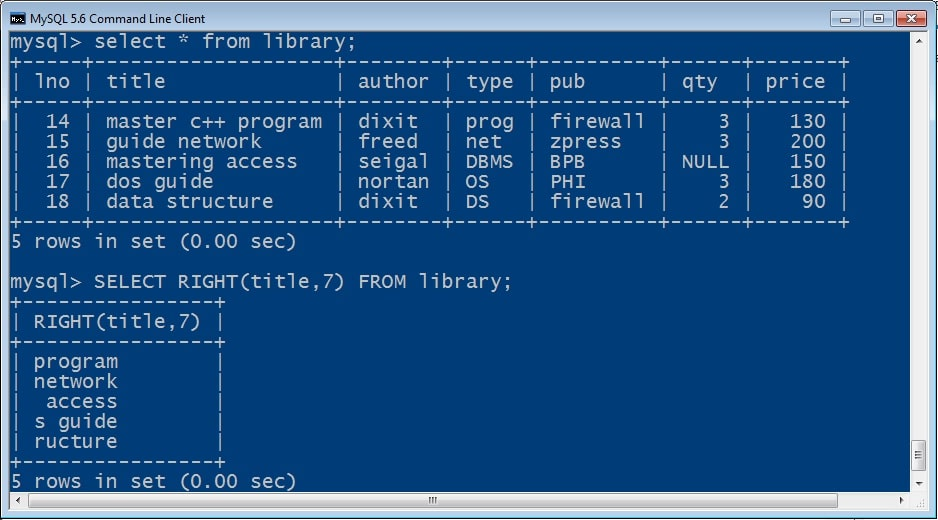Example 2:

SUBSTR()
It retrieves first occurrence of specific part of given string starting at the specified character to the number of character specified.
Syntax:
MID(String/fieldname(s), Start_index, Length)
Substr(String/fieldname(s), Start_index, Length)
Substring(String/fieldname(s), Start_index, Length)
Example 1: Find the output for ‘SELECT MID(title, 13) FROM library WHERE qty <=2;’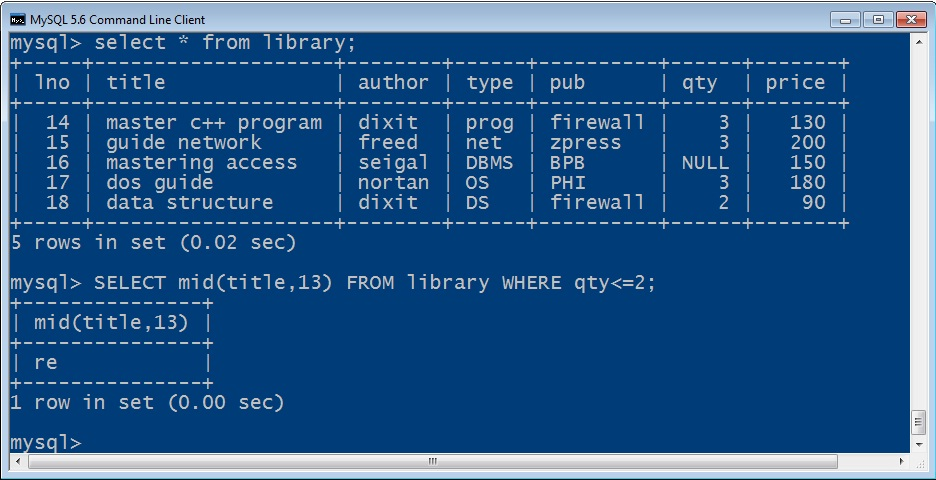Example 2: Display output of query: SELECT mid(‘techtipnow computer education’,11,9);INSTR()
It returns position of first occurrence of the substring from given string data.
Syntax:
INSTR(String/fieldname(s), substring)
Example 1: For given string ‘techtipnow computer education’. Write SQL command to display the position of ‘education’.TRIM()
It returns string after removing leading and trailing spaces from given string data.
Syntax:
TRIM(String/fieldname(s))
Example 1:RTRIM()
It returns string after removing trailing  spaces from given string data.
Syntax:
RTRIM(String/fieldname(s))
Example 1:
Example 2:

LTRIM()
It returns string after removing leading  spaces from given string data.
Syntax:
LTRIM(String/fieldname(s))
Example 1:
Example 2:

#### Date Functions

SQL provides various built in date and time functions to retrieve and convert specific part of chronological data. following are the date functions which have been discussed in the chapter as per syllabus:

DATE()
It extract and display date part of a given date-time expression.
Syntax:
DATE( Date/Datetime Expression)
Example 1:
Example 2:

NOW()
It display current date and time.
Syntax:
NOW()
Example 1:
Example 2:

MONTH()
It display Month as number of date passed.
Syntax:
MONTH(date/datetime expression)
Example 1:
Example 2:

MONTHNAME()
It display name of month of date passed.
Syntax:
MONTHNAME(date/datetime expression)
Example 1:
Example 2:

YEAR()
It extract and display year part of given date.
Syntax:
YEAR(date/datetime expression)
Example 1:
Example 2:

DAY()
It extract and display day part as number of given date.
Syntax:
DAY(date/datetime expression)
Example 1:
Example 2:

DAYNAME()
It extract and display name of day of given date.
Syntax:
DAYNAME(date/datetime expression)
Example 1:
Example 2:

CURDATE()
It returns current date.
Syntax:
CURDATE()
CURRENT_DATE()
CURRENT_DATE
Example 1:
Example 2:

#### Aggregate Functions

• Also known as Group Functions or Multiple Row Functions.
• helps to summarize large volume of data
• Returns single value for entire table or set of rows
• only one column can be specified within parenthesis of aggregate function.
• multiple aggregate functions can be used together in a SQL Query by separating them using comma.

Following are the aggregate functions which have discussed:

SUM()
It calculate and returns sum of given set of rows of a column.
Syntax:
SUM(DISTINCT | ALL set of values/column)
Example 1:
Example 2:

AVG()
It calculate and returns average/mean value of given set of rows of a column.
Syntax:
AVG(DISTINCT | ALL set of values/column)
Example 1:
Example 2:

COUNT()
It count and returns the given set of rows (excluding NULL) of a column.
Syntax:
COUNT(DISTINCT | ALL set of values/column)
Example 1:
Example 2:

COUNT(*)
It count and returns the given set of rows (including NULL) of a Table.
Syntax:
COUNT(*)
Example 1:
Example 2:

MAX()
It returns largest value from given set of rows of a column.
Syntax:
MAX(DISTINCT | ALL set of values/column)
Example 1:
Example 2:

MIN()
It returns smallest value from given set of rows of a column.
Syntax:
MIN(DISTINCT | ALL set of values/column)
Example 1:
Example 2:

### 2 thoughts on “Class 12 IP Database Query Using SQL Notes | IP Class 12 SQL Notes”

1.thankyou sir

error: Content is protected !!